MATHEMATICS
INTRODUCTION

Although Mathematics is a compulsory subject for GCSE, we hope that students will also enjoy the subject and gain much benefit from the course. Very many of the students will also wish to continue their study of Mathematics in the Sixth Form.  Mathematics is a versatile subject because it complements many others, as well as being a wonderful subject in its own right.

EXAM BOARD EDEXCEL
STRUCTURE OF
COURSE
UNIT:1                                        UNIT:3

Number                                      Ratio, Proportion and rates of change
UNIT: 2                       UNIT: 4                                       UNIT: 5
Algebra                          Geometry and measures         Statistics & probability
ASSESSMENT

Students will sit three exams; all units are assessed in each of the 3 papers.

The 3 papers will be equally weighted. 80 marks will be available for each and each will be 90 minutes long.

Paper 1 will be non-calculator. In papers 2 & 3 students may use a calculator

CONTENT

UNIT: 1

Topics in number include: factors, multiples, primes, standard form, surds, indices, fractions, percentages, decimals, rounding and accuracy

UNIT: 2 Topics in algebra include: algebraic manipulation, substitution, formulae, equations, identities, changing the subject, graphs, solving equations, inequalities, quadratics and sequences
UNIT: 3 Topics in ratio, proportion and rates of change topic include: standard units, scale factors, ratio, direct proportion, inverse proportion, compound units, gradient, growth and decay.
UNIT: 4 Topics in Geometry and measure include: describing shapes, constructions, angles, congruence, similarity, circle theorems, geometrical problems, 3D shapes, area, volume, Pythagoras, trigonometry and vectors
UNIT: 5 Topics in statistics and probability include: theoretical probability, experimental probability, Venn diagrams, sample spaces, tree diagrams, sampling, constructing tables, charts and diagrams, time series, interpreting and comparing data.

The content of the units is approximately split as follows

• Number (15%) , Algebra (30%),  Ratio, proportion and rates of change (20%)
• Geometry and measures (20%) Statistics and probability (15%)

The following will also be assessed within these units in the approximately stated proportions:

• Use and apply standard techniques (40%)
• Reason, interpret and communicate mathematically (30%)
• Solve problems within mathematics and in other contexts (30%)

It is expected that all students will sit the higher tier papers

•••••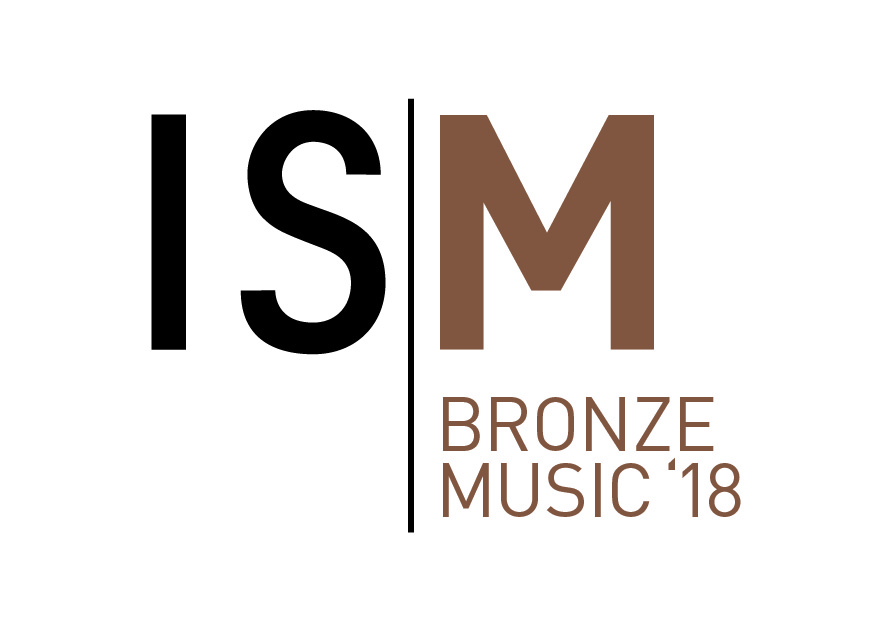••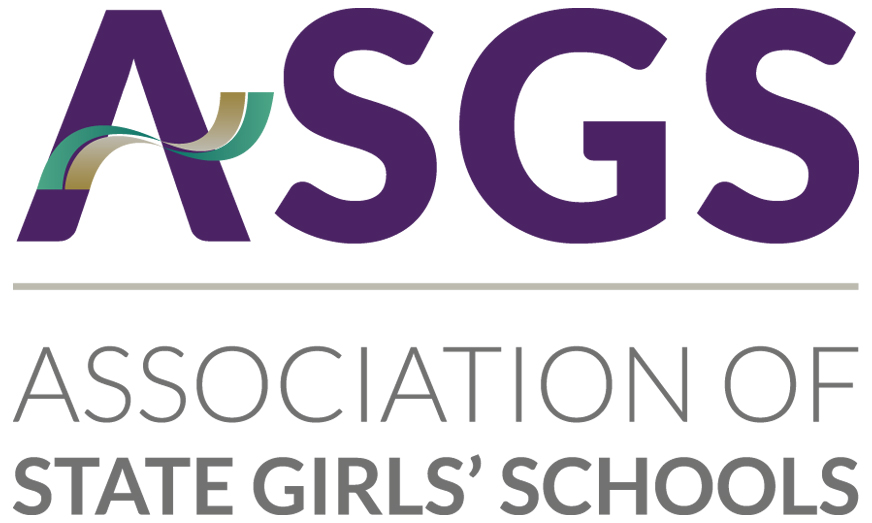•••••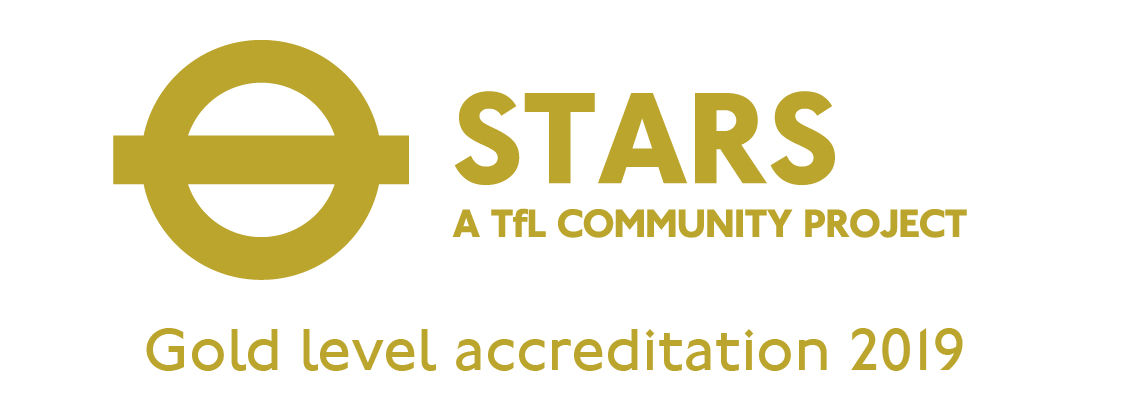•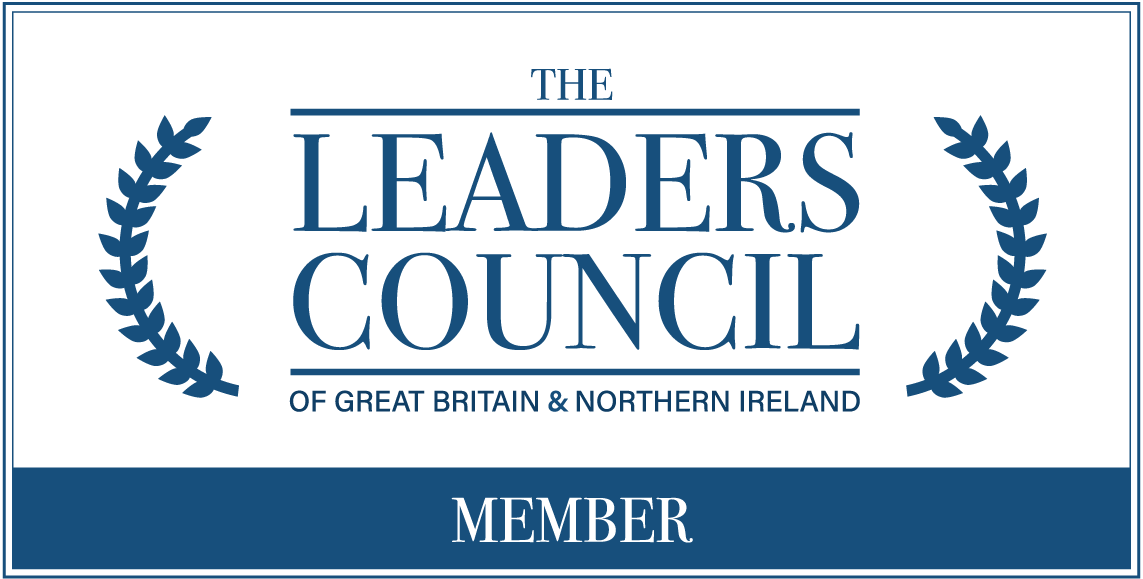•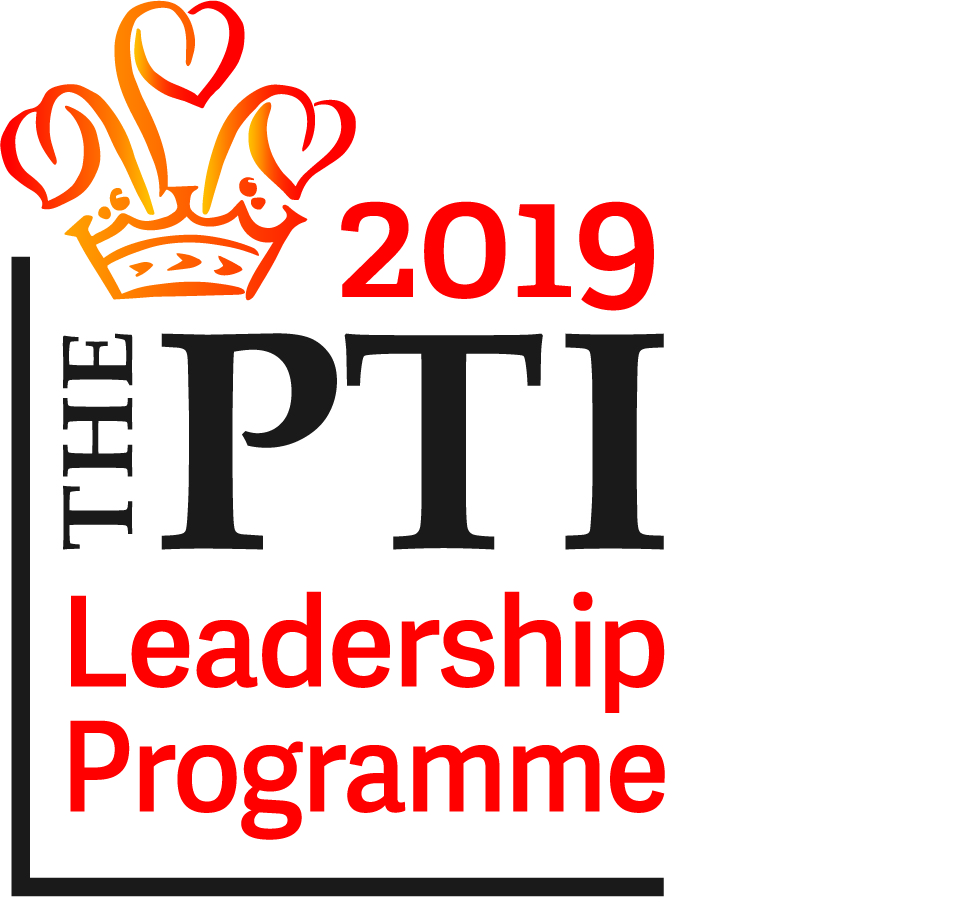•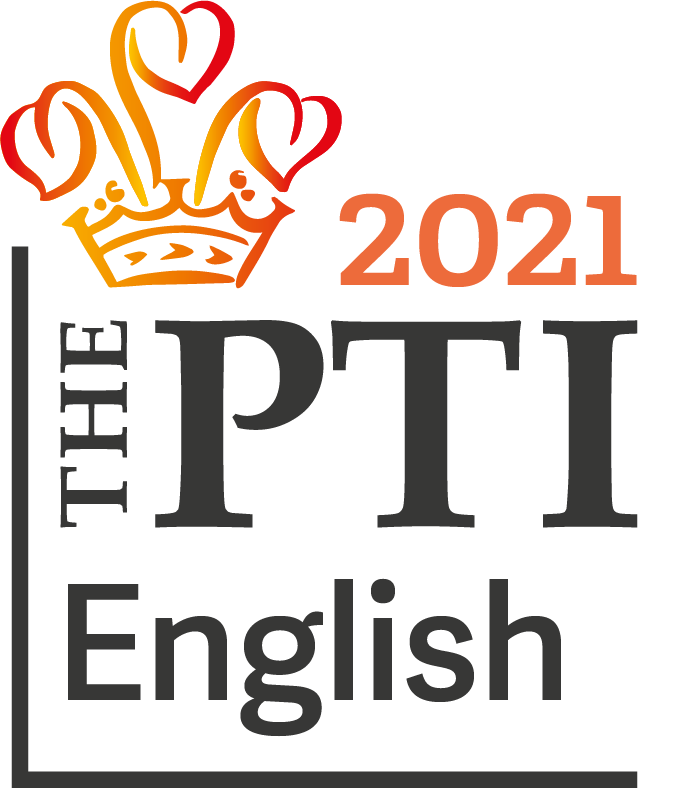•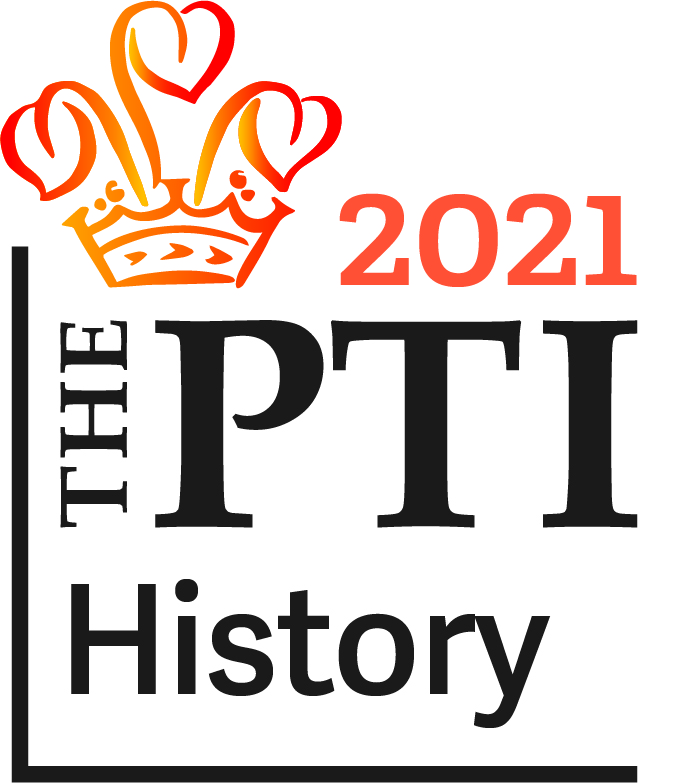•